# Derivative of trigonometric functions - Derivatives

## Derivative of trig functions

Before we start learning how to take derivative of trig functions, why don't we go back to the basics? Going back and reviewing the basics is always a good thing. This is because a lot of people tend to forget about the properties of trigonometric functions. In addition, forgetting certain trig properties, identities, and trig rules would make certain questions in Calculus even more difficult to solve. Let's first take a look at the six trigonometric functions.

## The 6 Trigonometric Functions

The first trigonometric function we will be looking at is $f(x) = \sin x$. If we are to graph the function, we will get this: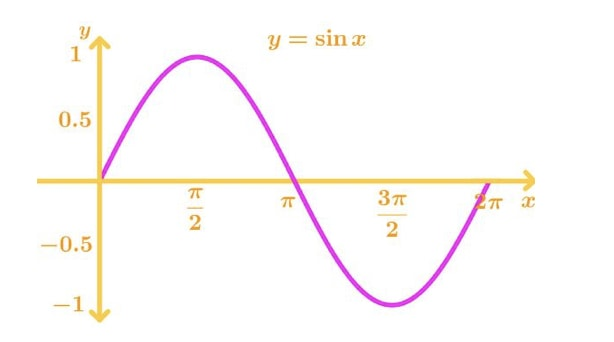Graph 1: sinx

Notice that the function is continuous from [-$\infty, \infty$], and it's a nice smooth curve with no sharp turns. This means that $\sin x$ is differentiable at every point, and so we will not have to worry about getting something undefined. In addition, we know that the slope at $x = \frac{\pi}{2} \pm \pi n, n \in I$ are $0$. Hence, the derivative of $\sin x$ will always be zero at those points.

Next is the function $f(x) = \cos x$. If we were graph the function, we will get: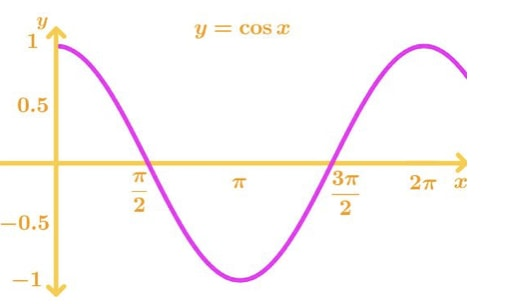Graph 2: cosx

Again note that the function is continuous from [-$\infty, \infty$], and it has a nice smooth curve. So $\cos x$ is also differentiable at every point. In addition, the slope is equal to 0 at $x$ = 0 $\pm \pi n, n \in I$. Hence, the derivative will of $\cos x$ will be 0 at those points.

Now the function $y = \tan x$ is a bit more interesting. It can be rewritten as $y= \frac{\sin x}{\cos x}$, the graph of this function looks like this: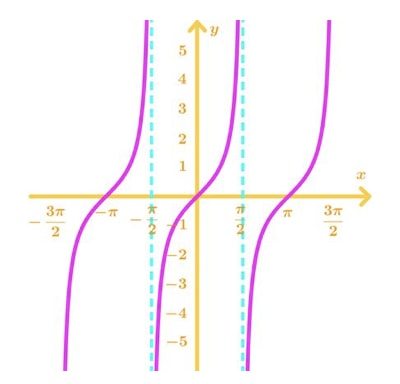Graph 3: tanx

Notice the $x$ values at $x = \frac{\pi}{2} \pm \pi n, n \in I$ are undefined, and have vertical asymptotes. This means the derivative of $\tan x$ will be not differentiable at those points. One interesting thing to note here is that the slope of $\tan x$ is never negative or 0. Hence, we should expect the derivative of $\tan x$ to always be positive.

Next are the reciprocal functions of sin cos tan. First, the reciprocal of $\sin x$ is $\csc x$. We see the graph of $f(x) = \csc x$ looks like this: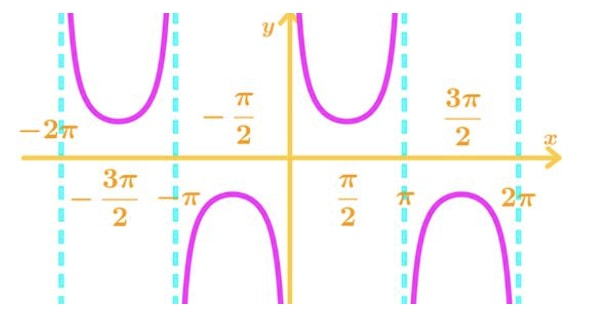Graph 4: cscx

Again, there are vertical asymptotes at $x$ = 0 $\pm \pi n, n \in I$. So they are not differentiable at those points. In addition, the slope of the function at $x = \frac{\pi}{2} \pm \pi n, n \in I$ are 0. So the derivatives are always 0 at those points.

Now the next function is $f(x) = \sec x$, which is the reciprocal of $\cos x$. Graphing gives: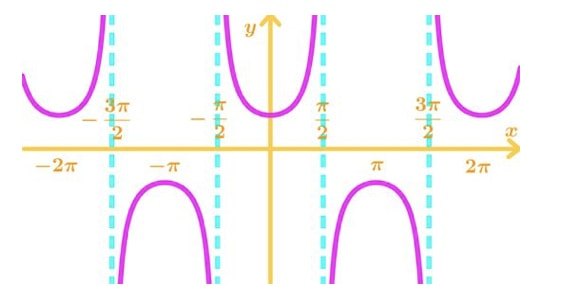Graph 5: secx

This is very similar to $\csc x$, but the difference is that the role's of the $x$ values has been switched. Now the $x$ values at $x = \frac{\pi}{2} \pm \pi n, n \in I$ are the vertical asymptotes and the $x$ value at $x = 0 \pm \pi n, n \in I$ are when the tangent slopes of the function are 0. Hence the derivative of the function is not differentiable at $x = \frac{\pi}{2} \pm \pi n, n \in I$ and the derivative is 0 at $x = 0 \pm \pi n, n \in I$.

The last function is $y = \cot x$.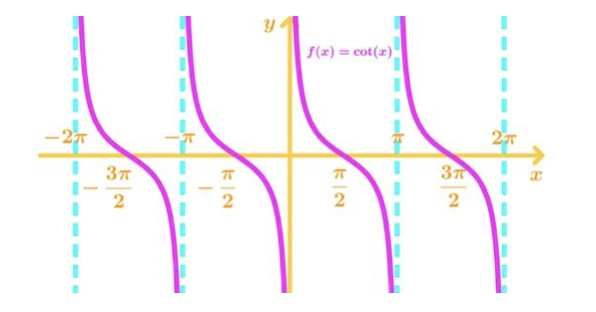Graph 6: cotx

Note that the vertical asymptotes are at $x = 0 \pm \pi n, n \in I$ and the slope of this never positive or 0. Hence, the derivative of this function is always positive, and not differentiable at $x = 0 \pm \pi n, n \in I$.

Now that we are finished looking at the 6 trig functions, let's now review some of the trigonometric identities that come in handy when taking derivatives.

## Trigonometric identities

The first six identities are reciprocal identities, which come in handy when you want your derivatives in a certain form.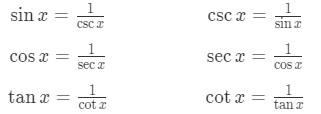Formula 1: Reciprocal identities

Now the most important identities are these three.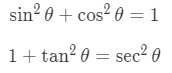Formula 2: Trigonometric identities

They are very useful when it comes to simplifying derivatives. Now that we've got the basics down, let's go ahead and actually take a look at the derivative of trig functions.

## Trig derivatives

The six trig function derivatives are as follows:

1. The derivative of $\sin x$ is: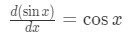Formula 3: Derivative of sinx

2. The derivative of $\cos x$ is: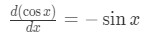Formula 4: Derivative of cosx

3. The derivative of $\tan x$ is: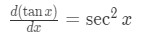Formula 5: Derivative of tanx

4. The derivative of $\csc x$ is: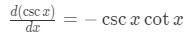Formula 6: Derivative of cscx

5. The derivative of $\sec x$ is: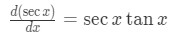Formula 7: Derivative of secx

6. The derivative of $\cot x$ is: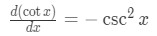Formula 8: Derivative of cotx

If you really want to know how we get the derivatives, then look at this article below:

Derivative of inverse trig functions

The article shows that the derivative of sin and cosine can be found using the definition of derivative, and the rest can be found with the quotient rule. Make sure you memorize these derivatives well! We will be using these to derive even harder trigonometric functions. You may also want to review the chain rule since a lot of hard trig derivatives require it.

## Derivative of sin^2x

We may know that the derivative of sin is $\cos x$, but what about the derivative of $\sin^{2} x$? Let's start with defining the function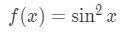Equation 1: Derivative of sin^2x pt.1

Let's move the square term so that the function becomes: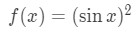Equation 1: Derivative of sin^2x pt.2

We are going to use the chain rule here. Recall that the chain rule states if you have a function within a function, call it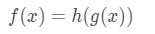Equation 1: Derivative of sin^2x pt.3

Then the derivative of this function will be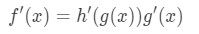Equation 1: Derivative of sin^2x pt.4

So if we set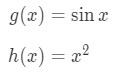Equation 1: Derivative of sin^2x pt.5

Then, the derivative of these functions will be: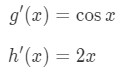Equation 1: Derivative of sin^2x pt.6

And so the derivative of the function f(x) will be: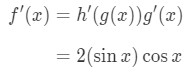Equation 1: Derivative of sin^2x pt.7

Which is the derivative of $\sin^{2} x$. It wasn't as hard as we thought! Let's look at around hard one.

## Derivative of cos^2x

Again, we know the derivative of cosine is $- \sin x$, but what about the derivative of $\cos^{2} x$? We set the function to be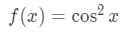Equation 2: Derivative of cos^2x pt.1

Again, if we rearrange the square in the function, then we will see that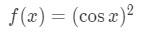Equation 2: Derivative of cos^2x pt.2

Using the chain rule again, we set: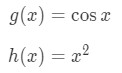Equation 2: Derivative of cos^2x pt.3

Taking the derivative of these functions gives: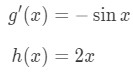Equation 2: Derivative of cos^2x pt.4

So the derivative of the function will be: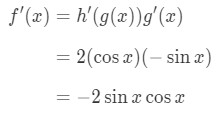Equation 2: Derivative of cos^2x pt.5

This is very similar to the derivative of $\sin^{2} x$, except we have an extra negative sign! Nevertheless, this is the derivative of $\cos^{2} x$. Let's try to find the derivative of another squared trigonometric function.

## Derivative of sec^2x

Again, the key idea of taking the derivative of $\sec^{2} x$ is using the chain rule. We define the function to be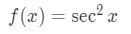Equation 3: Derivative of sec^2x pt.1

Rearranging the square in the function gives us: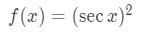Equation 3: Derivative of sec^2x pt.2

We set: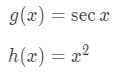Equation 3: Derivative of sec^2x pt.3

Taking the derivative of secant and $x^{2}$ gives: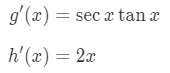Equation 3: Derivative of sec^2x pt.4

Again in case you forgot, the derivative of sec is $\sec x \tan x$. Hence, the derivative of $\sec^{2} x$ is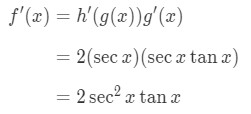Equation 3: Derivative of sec^2x pt.5

You should know by now that the procedure in taking the derivative of $\csc^{2} , \tan^{2}$, and $\cot^{2}$ are the same. So let's take a look at other trigonometric derivatives.

## Derivative of sinx^2

Now before you get confused, this is a function that looks similar to $\sin^{2} x$. However, $\sin^{2} x$ is different from $\sin x^{2}$. So of course, there derivatives will also be different. We define the function to be: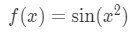Equation 4: Derivative of sinx^2 pt.1

Note that we will use the chain rule here. We set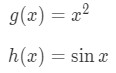Equation 4: Derivative of sinx^2 pt.2

We know the derivative of g(x) is 2x and the derivative of $\sin x$ is $\cos x$. So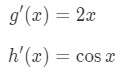Equation 4: Derivative of sinx^2 pt.3

Hence the derivative of the function will be: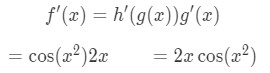Equation 4: Derivative of sinx^2 pt.4

Now let's try the same thing for finding the derivative of $\cos x^{2}$!

## Derivative of cosx^2

We define the function to be: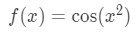Equation 5: Derivative of cosx^2 pt.1

Using the chain rule we set: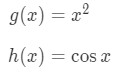Equation 5: Derivative of cosx^2 pt.2

Taking the derivative of g(x) gives 2x and taking the derivative of cosine gives $- \sin x$. So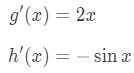Equation 5: Derivative of cosx^2 pt.3

Hence the derivative of the function is: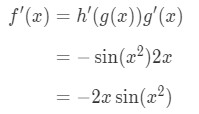Equation 5: Derivative of cosx^2 pt.4

Let's do two more before we start doing even harder trigonometric functions.

## Derivative of tanx^2

Again, the function we have is: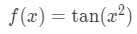Equation 6: Derivative of tanx^2 pt.1

Using the chain rule, we set g(x) and h(x) to be: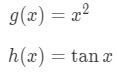Equation 6: Derivative of tanx^2 pt.2

The derivative of $x^{2}$ is 2x, and the derivative of $\tan$ is $\sec^{2}$, so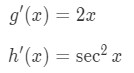Equation 6: Derivative of tanx^2 pt.3

Using the chain rule formula, we get the derivative of tanx^2 is: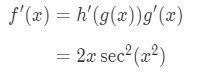Equation 6: Derivative of tanx^2 pt.4

This one is a bit harder because the derivative seems to have two squares; one from secant and one from x. Lastly, let's look at the derivative of $\csc x^{2}$.

## Derivative of cscx^2

The function we have is: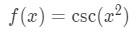Equation 7: Derivative of csc^2 pt.1

In order to do chain rule, we let two functions, g(x) and h(x), to be: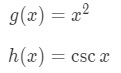Equation 7: Derivative of csc^2 pt.2

The derivative of $x^{2}$ is 2x, and the derivative of csc is -$\csc x \cot x$, so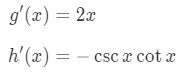Equation 7: Derivative of csc^2 pt.3

Using the chain rule formula, we get that the derivative of $\csc x^{2}$ is: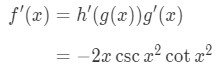Equation 7: Derivative of csc^2 pt.4
Again, the process in take the derivative of $\sec x^{2}$and $\cot x^{2}$ will also be the same. If you want to look at more questions of deriving trigonometric functions, then I suggest you look at this link.

http://tutorial.math.lamar.edu/Classes/CalcI/DiffTrigFcns.aspx

Now it's time move on and take a look at some questions which have applications to the slope of a function.

## Slope of a trigonometric function

Suppose we want to find the slope of the function: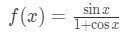Equation 8: Derivative slope of function pt.1

At the point x=0. How would we do it? Well, realize take find the slope of a function is just the same as taking the derivative. This will require using the quotient rule since the function is a quotient.

Recall that the quotient rule says the following:

If you have a function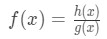Equation 8: Derivative slope of function pt.2

Then the derivative of this function will be: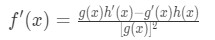Equation 8: Derivative slope of function pt.3

So if we were to set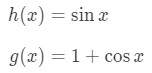Equation 8: Derivative slope of function pt.4

Then their derivatives will be: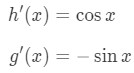Equation 8: Derivative slope of function pt.5

Hence, using the quotient rule gives the derivative: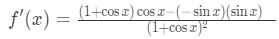Equation 8: Derivative slope of function pt.6

Simplifying this equation gives: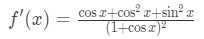Equation 8: Derivative slope of function pt.7

To simplify this equation, we would want to use the trigonometric identity: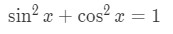Equation 8: Derivative slope of function pt.8

So our equation becomes: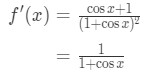Equation 8: Derivative slope of function pt.9

Now that we have the derivative, all we have to do is plug in the point x=0 to get the slope. Hence,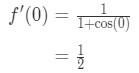Equation 8: Derivative slope of function pt.10

Thus, the slope of the function at the point x = 0 is exactly $\frac{1}{2}$. However, what if we were given the tangent slope of a function at a specific point, and we need to find that point?

## Finding the points given the slope

Suppose you are given that the function is: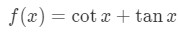Equation 9: Finding point given derivative slope pt.1

And you want to find the set of points which the slope of the tangent line is equal to 0. This means we need to take the derivative and set it equal to 0 to find the x values. Note that the derivative of $\tan$ is $\sec^{2}$ and the derivative of $\cot$ is –$\csc^{2}$, so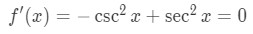Equation 9: Finding point given derivative slope pt.2

Note that the reciprocal identities state that: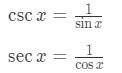Equation 9: Finding point given derivative slope pt.3

Hence, our equation becomes: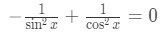Equation 9: Finding point given derivative slope pt.4

Let's try to solve for x in this equation. See that: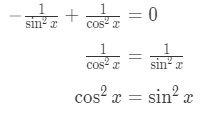Equation 9: Finding point given derivative slope pt.5

Moving the $\sin^{2} x$ to the other side gives: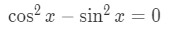Equation 9: Finding point given derivative slope pt.6

Recall the trigonometric identityEquation 9: Finding point given derivative slope pt.7

We can actually isolate $\sin^{2}$ by itself in the equation so that: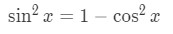Equation 9: Finding point given derivative slope pt.8

Substituting this into our equation gives: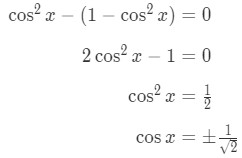Equation 9: Finding point given derivative slope pt.9

Now we need to look at the positive case and then the negative case. For the positive case we have: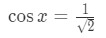Equation 9: Finding point given derivative slope pt.10

See that since x is not bounded, then were are infinitely many solutions. In fact, we know the solutions are: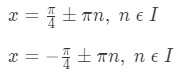Equation 9: Finding point given derivative slope pt.11

Now if we were to look at the negative case, then we have: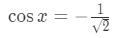Equation 9: Finding point given derivative slope pt.12

Again, there are infinitely many solutions for this. The solutions for this equation will be: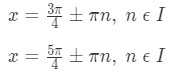Equation 9: Finding point given derivative slope pt.13

Now let's take a look at the four solutions as a whole. Notice that the two pairs are the same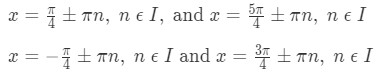Equation 9: Finding point given derivative slope pt.14

So we can just exclude the two solutions and say that the solutions areEquation 9: Finding point given derivative slope pt.15

So we can conclude that these are the set of points in which the slope of the function is equal to 0.

## Derivative of Inverse Trigonometric Functions

Now the Derivative of inverse trig functions are a little bit uglier to memorize. Note that we tend to use the prefix "arc" instead of the power of -1 so that they do not get confused with reciprocal trig functions. Regardless, they mean the same thing. For example, derivative of arctan is the same as the derivative of $\tan^{-1}$.

Here are the inverse trig derivatives that you will need to know.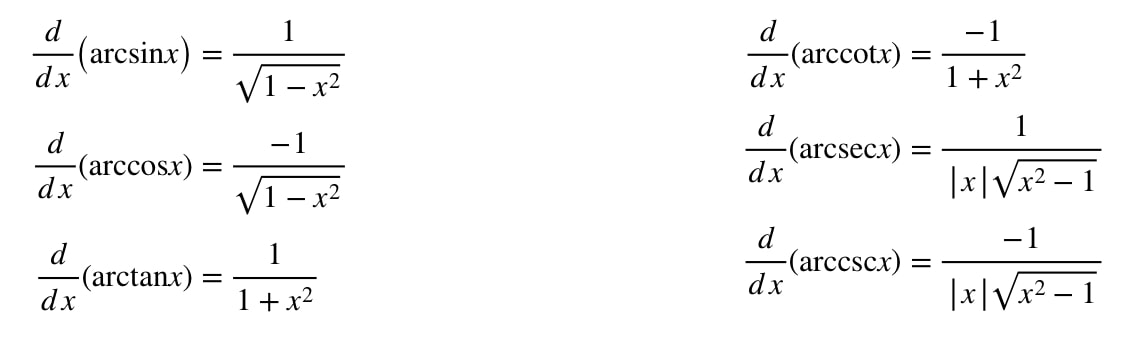Formula 9: Derivative of inverse trig functions Table

Note that the arctan derivative is similar to the derivative of arccot, except there is an extra negative sign. Coincidently, we see that the derivative of arcsin is similar to the derivative of arccos. The difference again is the negative sign. We will not be doing any examples of Derivative of inverse trig functions here. However if you are interested, then please look at this article here:

Derivative of inverse trig functions

The article here gives a detailed step-by-step solution in deriving these derivatives.

### Derivative of trigonometric functions

In this section, we will cover the six differential rules for trigonometric functions. In addition, continuing with the "Bracket Technique", we will integrate the differential rules for trig functions with the Chain Rule. As a memory trick, the derivative of any trig functions starting with "co-", such as cosine, cotangent and cosecant will be negative.

#### Lessons

###### Differential Rules - Trigonometric Functions$\frac{{d}}{{{d}x}}\;\sin x = \cos x$$\frac{{d}}{{{d}x}}\;\cos x = - \sin x$$\frac{{d}}{{{d}x}}\;\tan x = {\sec ^2}x$$\frac{{d}}{{{d}x}}{\;cot}x = - {\csc ^2}x$$\frac{{d}}{{{d}x}}\;\sec x = \sec x{\;tan}x$$\frac{{d}}{{{d}x}}\;\csc x = - \csc x\;\cot x$